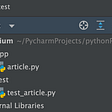# np arange with Example — pythonpip.com

In this python tutorial. we will learn how to use NumPy arrange method. The np.arange() method builds a very basic array based on a user-supplied numerical range.

NumPy is a Python library that is commonly regarded as the most significant for numerical computation. The np array is used to generate numerical ranges.

# Difference between inbuilt range and np.arrange() Method

Both functions accept the start, stop, and step arguments, which is one important commonality. However, range() has an important limitation — it can only work with integers! If you pass in any other data type, you will get a TypeError.

The Syntax:The parameters are:

• start: This is the number (integer or decimal) that defines the first value in the array.
• stop: This is the number that defines the end of the array and isn’t included in the array.
• step: This is the number that defines the spacing (difference) between each two consecutive values in the array and defaults to 1.
• dtype: This is the type of the elements of the output array and defaults to None.

`import numpy as np` `numpy.arange([start, ]stop, [step, ], dtype=None) -> numpy.ndarray`

How To Import NumPy You must first import the NumPy library into your Python script before using the `np.arange()` method. This can be done with the following code:

# Simple Example Using np arrange

Output:

The sample code to generate some numerical array:

`np.arange(0,6) np.arange(-3,2) np.arange(0,0)array([0, 1, 2, 3, 4, 5, 6]) array([-3, -2, -1, 0, 1, 2]) array([], dtype=int64)`

# np.arange() method using a single argument

Output:

Let’s create a python array using np.arrange() by passing a single parameter:

`np.arange(1) np.arange(5)array() array([0, 1, 2, 3, 4])`

# np.arange() Method’s step Argument

`np.arange(0,10, step=5)` Output:

The np.arange() method in NumPy takes an optional third argument, step, which specifies how much space should be between each element of the array it returns. The `step` is set to one by default.

Let’s create an array that had a space of 5 integers between them, we could specify step=5:

`array([0, 5])`

Originally published at https://www.pythonpip.com on March 26, 2022.

--

--

--

## More from Parvez Alam

Hey, I am Parvez Alam. A software developer since 2009. I am a programming addict. I love learning and sharing knowledge. https://www.phpflow.com/

Love podcasts or audiobooks? Learn on the go with our new app.

## Is no-code really the future of development?## Step-by-step guide to setting up Prometheus Alertmanager with Slack, PagerDuty, and Gmail## Similarities between coding and our way of being## Beginner’s Guide to Setting up a Tezos Node with Docker, Opam and Source with Common Problems and…## Failing fast but learning faster## Contrasting AWS ECS and Kubernetes## Daily SCRUM — Not a status meeting## Parvez Alam

Hey, I am Parvez Alam. A software developer since 2009. I am a programming addict. I love learning and sharing knowledge. https://www.phpflow.com/

## MySQL SUM() function## Introducing the unit testing in Python## Checking your classes using automated unit test in Python